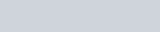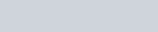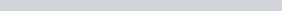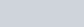•
•
•
•
•
•
•
•

# LCM AND HCF PROBLEMS TRICKS

In today’s article, we are going to talk about some important arithmetic formulae on LCM and HCF, these formulae may be helpful to you, in all types of competitive exams.1). To find the HCF of two numbers.
Method

Split the given numbers into prime factors and then find the product of all the prime factors, which are common to all the numbers. The product is the required HCF.

2). To find the HCF of decimals.
Method-

First, make the same numbers of decimals places in all the numbers, and then see the numbers as integer and find the HCF, and in the answer put the decimal places as there in each of the numbers.

3). To find the LCM of two numbers.
Method-

Split the numbers into prime factors and then find the product of the highest power of all the factors that occurred in given numbers. The product will be the required LCM.

4). To find the LCM of the decimals.
Method-

First, make sure the same numbers of decimals places in all the numbers. then find the LCM as they are integers, and then in the answer put the decimal places as there in each of the numbers.

5). HCF of the fraction6). LCM of the fraction7). Product of the numbers8). The greatest number that will exactly divide x,y, and z is =HCF of x, y, and z

9). The greatest number that will divide the numbers x,y, and z such that they leave remainder a,b and c respectively = HCF of (x-a),(y-b),(z-c)

10). The least number which exactly divisible by the numbers x,y, and z is =  LCM of x,y, and z

11). The least number which when divided by x, y, and z leaves remainder a,b, and c respectively, the special features are always observed that (x-a)=(y-b)=(z-c)=k
Then the least number is = (LCM of x, y, and z)-K

12). The least number which when divided by the numbers x y and z leaves the same remainder r in each case is = (LCM of x, y, and z)+r

13). The greatest number that will divide x y and z leaving the same remainder r in each case = HCF of (x-r),(y-r),(z-r)

14). The greatest number that will divide x y and z leaving the same remainder in each numbers is = HCF of |(x-y)|, |(y-z)|, |(z-x)|

15). To find the n digit greatest number which when divided by x y and z, leaves no remainder.

The following steps can be taken:

Step1: find LCM of x y and z that is L
Step2: divide n digit the greatest number by L and find remainder R
Step3: the required number is= n digit greatest number -R

16). To find the n digit smallest number which when divided by x y and z leaves no remainder.

The following steps can be taken:

Step1: find LCM of x y and z, i.e. L
Step2: divide the n digit the smallest number by L and find the remainder R.
Step3: the required number is= n digit smallest number + (L-R)

17). If there are n numbers and HCF of each pair is x and LCM of all the n numbers is y the product of the numbers is-18). In the question of the bell, if the ringing interval is given in seconds, minutes, and hours, then the time in which all bells rang at the same time is = (LCM of time) + initial time.